# Triangle Areas - Basic Calculations

Triangle Areas - Basic Calculations
Go back to  'Triangles'

Given any triangle, let us call one of its sides the base of the triangle. Then, the perpendicular dropped from the opposite vertex onto the base will be called the height (or altitude) of the triangle. In the following figure, BC has been taken to be the base of $$\Delta ABC$$, while AD is the height: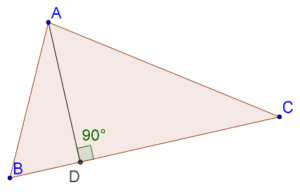The area of this triangle will be:

Area = ½ × BC × AD

Of course, we could have taken any of the other two sides and the base, but the height would then have changed accordingly. The final value of the area will (obviously) come out to be the same in each case.

Can you recall how this formula for the area was derived? It was derived from the relation for the area of a parallelogram. In the figure above, if you complete the parallelogram ABCE, the area of $$\Delta ABC$$ will be exactly half of parallelogram ABCE (why?), and we saw (in an earlier chapter) how the area of a parallelogram can be written as a product of its base and height: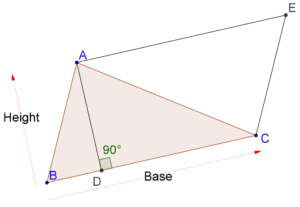Example 1: Consider a triangle ABC which is right-angled at B. Let AC = 5 cm, and AB = 4 cm. What is the area of this triangle?

Solution: Consider the following figure: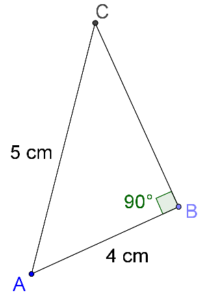By the Pythagoras Theorem,

BC2 = AC2 - AB2 = 52 - 42

è BC2 = 25 - 16 = 9

è BC = 3 cm

Now, if we take the base of this triangle to be AB, the height will be BC, and so:

Area = ½ × AB × BC = ½ × 4 ×3 = 6 cm2

Example 2: What is the area of an equilateral triangle in terms of its side(s)?

Solution: Recall that the sides of an equilateral triangle are equal. Let the length of each side be denoted by x: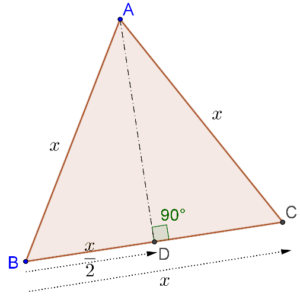Note that we have dropped the perpendicular AD from A onto BC. Clearly, BD = x/2. In $$\Delta ABD$$, let us apply the Pythagoras Theorem and find out the value of AD in terms of x:

è AD2 = x2 - (x/2)2 = x2 - x2/4

è AD = $$\left( {\sqrt 3 x} \right)/2$$

Now that we have AD, we can find the area of the triangle easily:

Area = ½ × BC × AD = ½ × x × $$\left( {\sqrt 3 x} \right)/2$$

è Area = $$\left( {\sqrt 3 /4} \right){x^2}$$

Example 3: Find the area of an isosceles triangle whose sides are 5 cm, 5 cm and 8 cm.

Solution: Consider the following figure: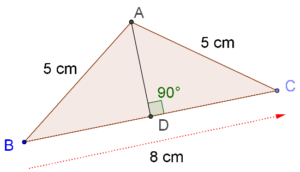We have:

AD2 = AB2 - BD2 = 52 - 42 = 9

Thus,

Area = ½ × BC × AD = ½ × 8 × 3 = 12 cm2

grade 9 | Questions Set 1